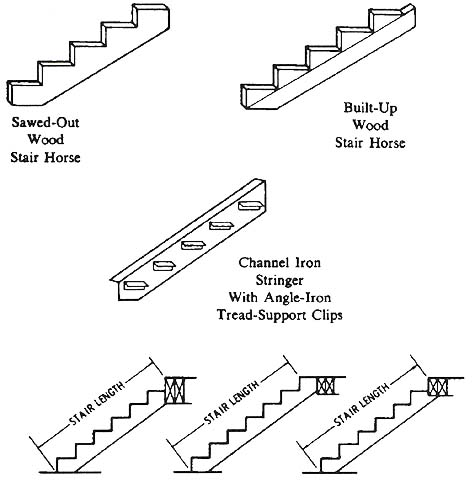# Stair Layout: Conditions and Dimensions

Conditions

A stair layout usually must comply with certain fixed conditions and/or specific dimensions, such as height, size of opening, available space, direction of run, etc. In addition, there are certain general conditions which the layout must meet, such as tread-rise dimension, tread-run dimension, etc.

While there is no standard tread-rise dimension as such, experience has shown that it should be in the area of 7 inches. Too large a rise results in a steep stairway, sometimes referred to as a "hard" stairway, because it is difficult both to ascend and descend. Also, the tread rise must be proportional to the tread run; that is, the tread run narrows as the tread rise increases and vice versa. There are numerous methods or rules to determine the dimensions of the tread rise and run. A very simple one, easy to remember and quite satisfactory for general use, is that the sum of the tread rise and run dimensions should total 17 inches. The tread rise dimension should be held as close as possible to the optimum 7 inches. Another rule in common use is that the run dimension plus twice the rise dimension should equal 24.

## Dimensions

Specific layout dimensions for stairs are determined from the given or known dimensions and/or conditions, such as size of opening, height from floorto floor, available space, direction of run, etc. The manner or method of layout, therefore, is dictated by the given or known information. For instance, the most frequently encountered situation is one where the total rise (distance from level to level) is known, and a set of straight stairs is to be installed. If space is not limited, all necessary dimensions for layout of a comfortable set of stairs maybe calculated from this one dimension.

## Example

A straight set of stairs is to be constructed and installed from one level to the level above. The vertical distance from floor-level to floor level is 12 feet 8 inches.

Total Rise: Vertical distance between levels 12' 8", or 104".

Tread Rise: The calculation of the tread rise is done in three steps. First, determine the approximate number of rises by dividing the total rise in inches by 7. Second, select a whole number that is close to the number calculated. This will be the number of rises or steps in the stairs. Third, calculate the tread rise dimension by dividing the total rise measurement by the number of rises or steps selected.

Using the example stated above, 7 will divided into 104 almost 15 times; therefore the selection is between 14 and 15. If 15 rises are selected, the tread rise will be 6 15/16". If 14 rises are selected, the treads rise will be 7 7/16".

Tread Run: The tread run is calculated by subtracting the tread rise dimension from 17. As this is an approximate figure, the actual tread run dimension is rounded off to the closest convenient fraction; for instance, for a 6 15/16" tread rise a 10" run maybe used, while for a 7 7/16," rise a 9 1/2" run would be proper.

Total Run: The total run is calculated by multiplying the tread run dimension by one less than the number of rises. Using the figures from the above example, the total run for 14 rises would be 123 1/2" (13 x 9 1/2), and the total run for 15 rises would be 140" (14 x 10).

The two examples stated above are illustrated in Fig. 1.Above: Fig. 1: 15 Rises (left) and 14 Rises (right)

Stairs are usually supported by either stair horses or by stair stringers. Stair horses are an underneath style of support member, with the stair tread resting on the steps of the horses. Stair stringers are side support members; the treads are located between the stringers and are supported by end attachment.

When constructing simple stairs, the stair horse style of construction is usually used with wood materials and the stair stringer style when structural steel materials are used ( Fig. 2 below).Home •  •

Top of page

Monday, 2008-12-29 17:02 PST